Mode Shape/Amplitude Plot PropertyManager

The Mode Shape/Amplitude Plot PropertyManager allows you to plot mode shapes for frequency, buckling, and linear dynamic studies.

To open the Mode Shape/Amplitude Plot PropertyManager, right-click Results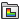and select Define Mode Shape/Amplitude Plot.

Display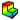Component Select a mode shape amplitude component to plot. Directions are based on the selected reference geometry. The mode shape amplitudes have no units and they do not depict actual displacements. Mode shapes illustrate the profile of the mode only (i.e., the displacement of nodes relative to each other).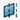Plane, Axis or Coordinate System Select a reference plane or a reference axis to plot mode shapes. Show as vector plot Generates a vector plot where a vector is plotted at each node to show the magnitude and direction of the selected component. You can control the size and density of the vectors in a vector plot using the Vector Plot Options PropertyManager. Available for directional displacement components only. Show plot only on selected entities Generates plots only for the selected entities. Normalize Mode Shape (max value = 1) Sets the maximum amplitude value to 1. All amplitude values are divided by the maximum absolute value.

Plot Step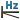Frequency Displays the frequency corresponding to the specified mode shape.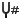Mode Shape Sets the mode shape number for which the amplitudes are plotted.

Deformed Shape

If checked, the selected component is plotted on the deformed shape of the model.

 Automatic Displays the default Scale Factor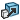that the program uses to scale the largest deformation to 10% of the largest dimension of the smallest box that surrounds the model. True scale Displays the actual deformed shape of the model (Scale Factor is 1.0). User defined Allows you to enter your own Scale Factor. In most cases, a larger scale factor helps to appreciate the deflection. Show colors Toggles the visibility of color gradient results on the deformed shape of the model.

Property

 Include title text Type a custom title for the plot. Associate plot with name view orientation Associate a predefined view orientation with the active plot.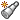Associate with current view Associates the current view with the active plot.